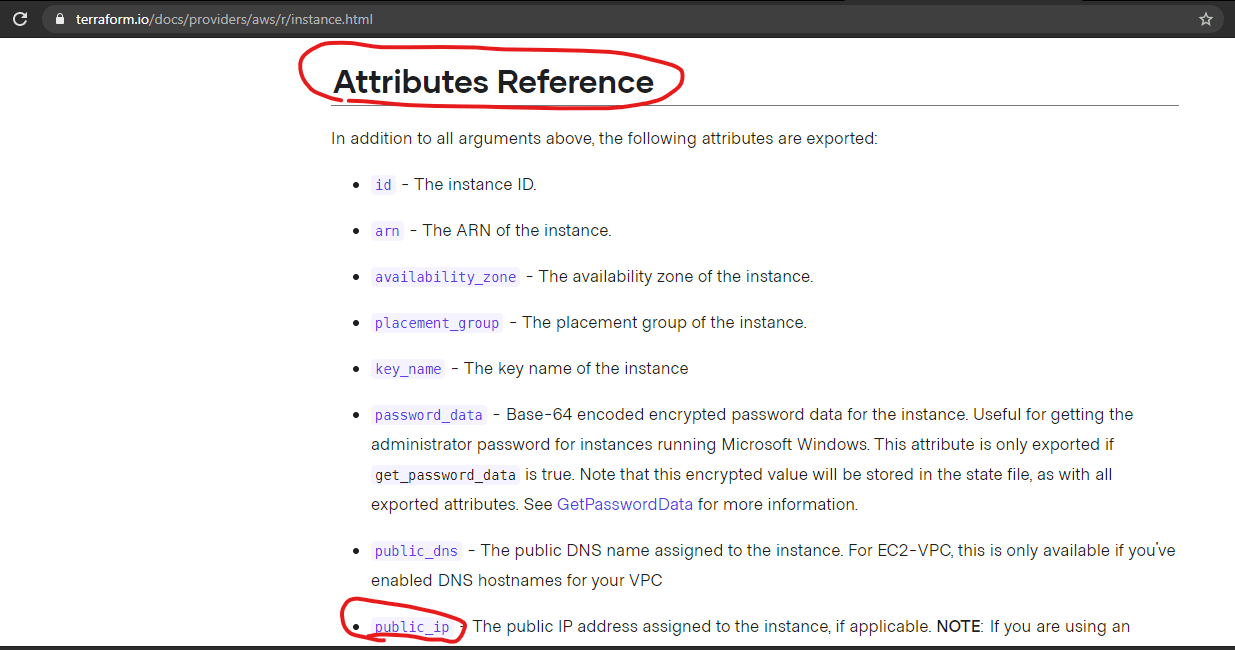### Types in Terraform Configuration Language

• Simple/Primitive Types:

• string
• number
• bool
• Complex Types

• Collection Types:
• list: list of any type. list(bool) is list of booleans, list => list(any)
• map
• set
• Structural Types:
• object
• tuple
• Dynamic Type: any

• Examples

• You can define your own object type for aws credentials sytnax is ` object( { access_key=string, secret_key=string, region=string } )` and the input would be
``````{
access_key 	= "jkashfkjdahfkajs"
secret_key	= "klsjdflkdsjdlafs"
region     	= "us-west-2"
}
``````
• Exampes with variables: All the variables can eb defined using any of the above types

``````variable "bucketname" {
type    = string
default = "yourbucketname.com"
}

variable "regions" {
type    = list(string),
default = ["us-west-1", "us-west-2", "us-east-1", "us-east-2" ]
}

variable "aws_credentials" {
type    = object( { access_key=string, secret_key=string, region=string } )
default = {
access_key 	= "jkashfkjdahfkajs"
secret_key	= "klsjdflkdsjdlafs"
region     	= "us-west-2"
}
}
``````
• Now show the output as public ip address to the user & for this we would navigate to Attribute section of the resource• The terraform template looks like
``````provider "aws" {

}

variable "securitygroupid" {
type    = string
}

variable "keyname" {
type    = string
}

resource "aws_instance" "firstec2" {
ami                             = "ami-003634241a8fcdec0"
instance_type                   = "t2.micro"
key_name                        = var.keyname
vpc_security_group_ids          = [ var.securitygroupid ]
tags                            = {
Name                        = "firstec2"
}
}

output "public-ip" {
value = aws_instance.firstec2.public_ip
}

output "private-ip" {
value = aws_instance.firstec2.private_ip
}

``````### Terraform resource dependencies

• Lets create ec2 machine, vpc and one s3 bucket, The order or creation should be
• vpc
• s3 bucket
• ec2 machine
• use depends_on in resources
``````provider "aws" {

}

variable "securitygroupid" {
type    = string
}

variable "keyname" {
type    = string
}

resource "aws_instance" "firstec2" {
ami                             = "ami-003634241a8fcdec0"
instance_type                   = "t2.micro"
key_name                        = var.keyname
vpc_security_group_ids          = [ var.securitygroupid ]
tags                            = {
Name                        = "firstec2"
}

depends_on                      = [ aws_s3_bucket.myfirsts3bucket]
}

resource "aws_vpc" "myfirstvpc" {
cidr_block                      = "192.168.0.0/16"

}

resource "aws_s3_bucket" "myfirsts3bucket" {
bucket                          = "skjdfhkjsah.com"

depends_on                      = [ aws_vpc.myfirstvpc ]

}

``````

### Create Multiple resources and give a different name

• Add three subnets to the vpc and names of the subnets should be taken from variable
• Use count to create multiple resources and count.index to know current iteration
``````variable "securitygroupid" {
type    = string
}

variable "keyname" {
type    = string
}

variable "subnetnames" {
type    = list(string)
default = [ "subnet1", "subnet2", "subnet3"]
}

variable "cidrrnages" {
type    = list(string)
default = ["192.168.0.0/24", "192.168.1.0/24", "192.168.2.0/24"]
}

resource "aws_instance" "firstec2" {
ami                             = "ami-003634241a8fcdec0"
instance_type                   = "t2.micro"
key_name                        = var.keyname
vpc_security_group_ids          = [ var.securitygroupid ]
tags                            = {
Name                        = "firstec2"
}

depends_on                      = [ aws_s3_bucket.myfirsts3bucket]
}

resource "aws_vpc" "myfirstvpc" {
cidr_block                      = "192.168.0.0/16"

}

resource "aws_s3_bucket" "myfirsts3bucket" {
bucket                          = "skjdfhkjsah.com"

depends_on                      = [ aws_vpc.myfirstvpc ]

}

resource "aws_subnet" "subnet" {
count                           = 3

vpc_id                          = aws_vpc.myfirstvpc.id
cidr_block                      = var.cidrrnages[count.index]
tags                            = {
Name                        = var.subnetnames[count.index]
}

depends_on                      = [ aws_vpc.myfirstvpc ]

}

``````

This site uses Akismet to reduce spam. Learn how your comment data is processed.